### Home > CALC > Chapter Ch10 > Lesson 10.2.2 > Problem10-102

10-102.
1. Determine if the following series converge or diverge. State the test you used. Homework Help ✎

1.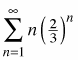2.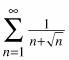3.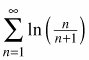4.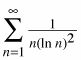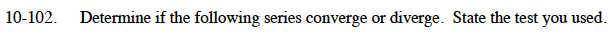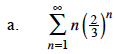The Ratio Test can be used.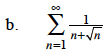$n+\sqrt{n}\le 2n$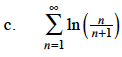$\ln\Big(\frac{n}{n+1}\Big)=\ln(n)-\ln(n+1)$

Write out some terms of the series. You should notice that this is a telescoping series.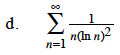What is the value of the first term of the series?